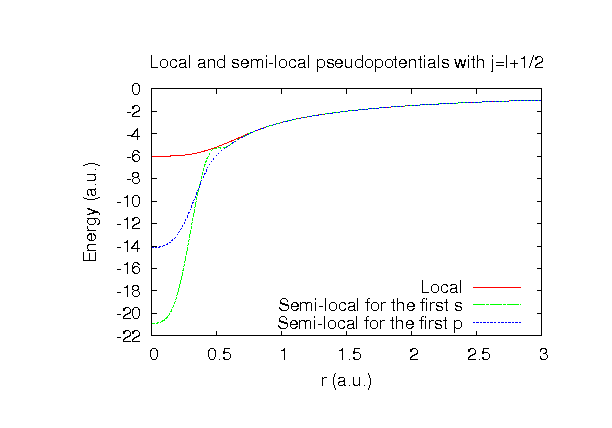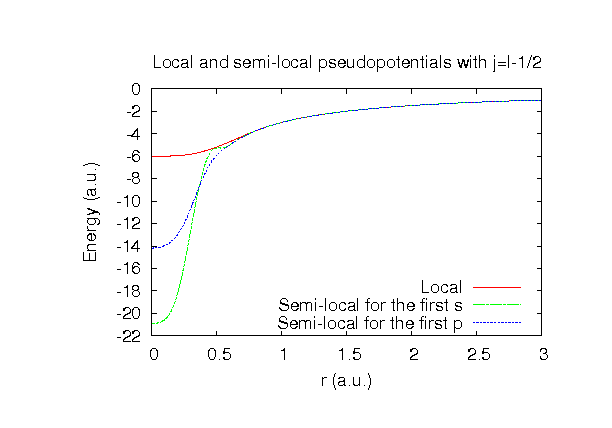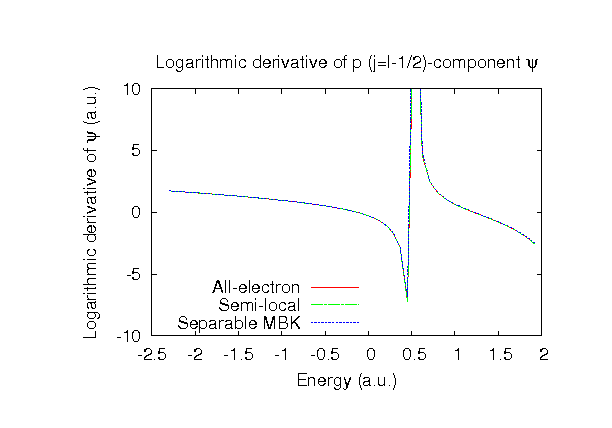### Fully relativistic pseudopotentials

Fully relativistic pseudopotentials generated by the MBK (PRB 47, 6728 (1993)) scheme within LDA (CA13) and GGA (PBE13) which contain a partial core correction and fully relativistic effects including spin-orbit coupling.

### Pseudo-atomic orbitals

The number below the symbol means a cutoff radius (a.u.) of the confinement potential. These file includes fifteen radial parts for each angular momentum quantum number l (=0,1,2,3). The basis functions were generated by variationally optimizing the corresponding primitive basis functions in the single atom, the dimer, and a LiO molecule. The input files used for the orbital optimization can be found at Li_opt.dat , Li2_opt.dat , and LiO_opt.dat . Since Li_CA13.vps and Li_PBE13.vps include the 1s and 2s states (3 electrons) as the valence states, the minimal basis set is Li*.*-s2. Our recommendation for the choice of cutoff radius of basis functions is that Li8.0.pao is enough for bulks, but Li10.0.pao or Li12.0.pao is preferable for molecular systems.

### Benchmark calculations by the PBE13 pseudopotential with the various basis functions

(1) Calculation of the total energy as a function of lattice constant in the bcc structure, where the total energy is plotted relative to the minimum energy for each case. a0 and B0 are the equilibrium lattice constant and bulk modulus obtained by fitting to the Murnaghan equation of state. The difference between Li8.0-s3p2 and Li8.0-s3p3d2 in the total energy at the minimum point is 0.013 eV/atom. An input file used for the OpenMX calculations can be found at Libcc-EvsV.dat . For comparison the result by the Wien2k code is also shown, where the calculation was performed by default setting in the Ver. 10.1 of Wien2k except for the use of RMT x KMAX of 12.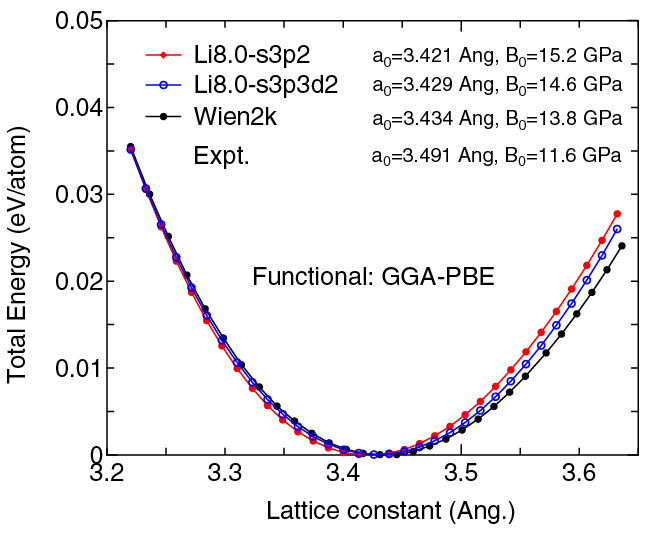(2) Calculations of the band dispersion in the bcc structure, where the non-spin polarized collinear calculation with the lattice constant of 3.491 Ang. was performed using Li_PBE13.vps, Li8.0-s3p2, and Li8.0-s3p3d2, and the origin of the energy is taken to be the Fermi level. The input file used for the OpenMX calculations can be found at Libcc-Band.dat . For comparison the result by the Wien2k code is also shown, where the calculation was performed by default setting in the Ver. 10.1 of Wien2k except for the use of RMT x KMAX of 12.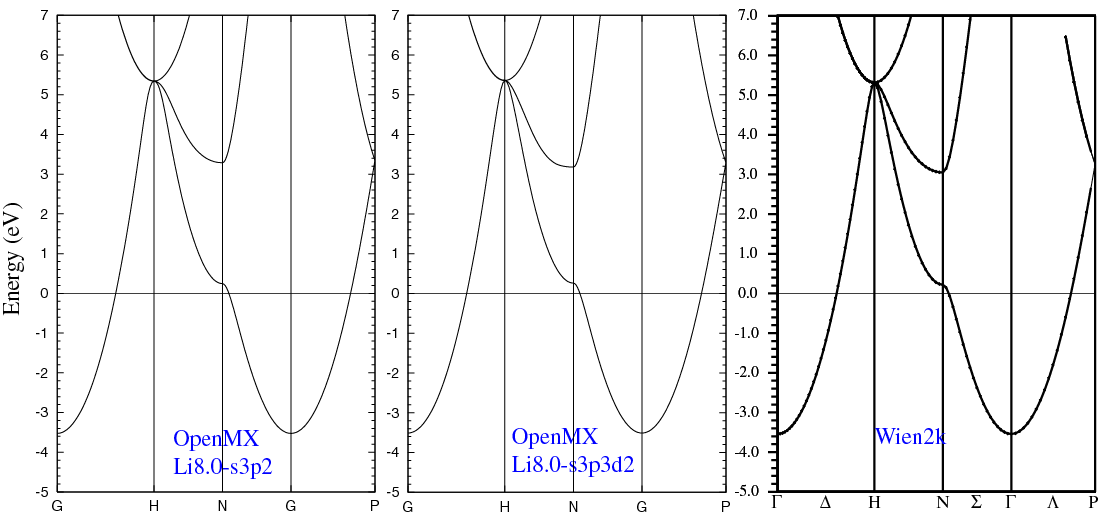(3) Calculations of the total energy as a function of lattice constant of LiCl in the rock salt structure, where the total energy is plotted relative to the minimum energy for each case. a0 and B0 are the equilibrium lattice constant and bulk modulus obtained by fitting to the Murnaghan equation of state. The difference between Cl7.0-s2p2d1 and Cl7.0-s3p3d2 in the total energy at the minimum point is 0.0112 eV/atom, where Li8.0-s3p2d1 was used as basis functions for Li for both the cases. An input file used for the OpenMX calculations can be found at LiCl-EvsV.dat . For comparison the result by the Wien2k code is also shown, where the calculation was performed by default setting in the Ver. 9.1 of Wien2k except for the use of RMT x KMAX of 12.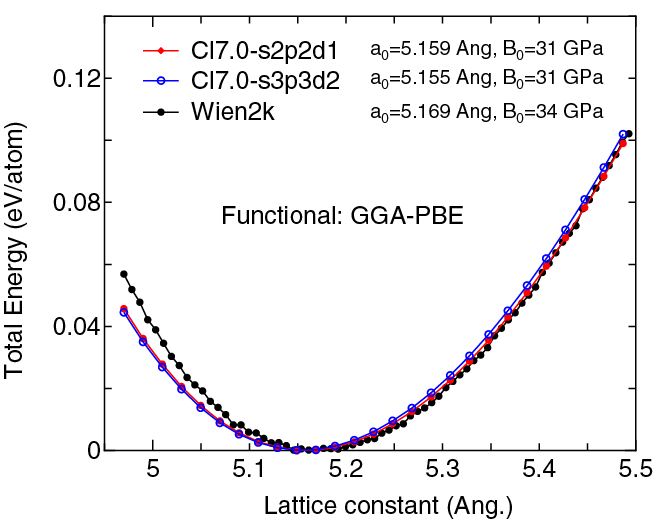(4) Calculations of the band dispersion of LiCl in the rock salt structure, where the non-spin polarized collinear calculation with the lattice constant of 5.106 Ang. was performed using Li_PBE.vps, Cl_PBE13.vps, Li8.0-s3p2d1, and Cl7.0-s2p2d1, and the origin of the energy is taken to be the top of the valence band. The input file used for the OpenMX calculations can be found at LiCl-Band.dat . For comparison the result by the Wien2k code is also shown, where the calculation was performed by default setting in the Ver. 9.1 of Wien2k except for the use of RMT x KMAX of 12.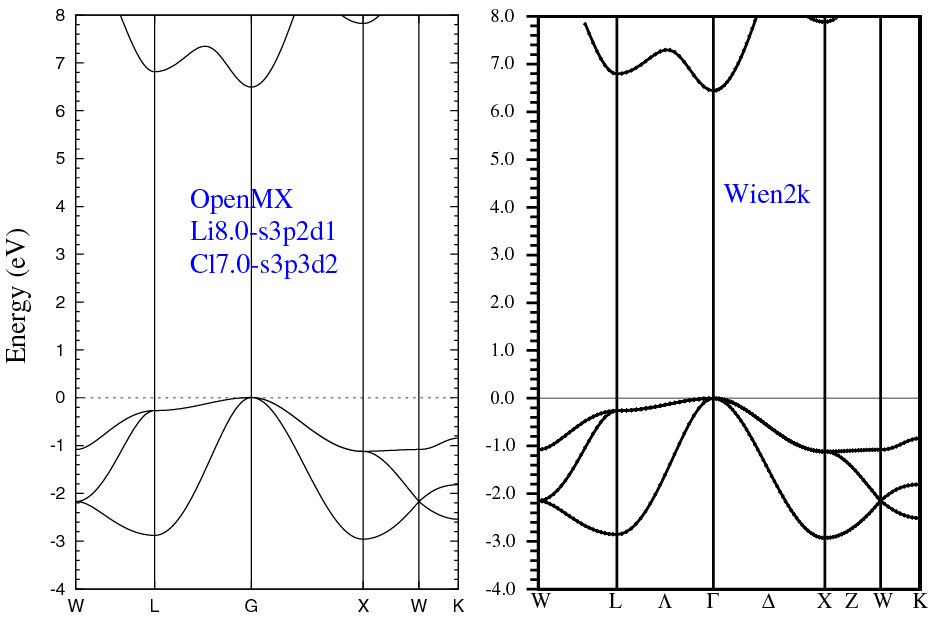(5) Calculations of a lithium dimer molecule, where Li_PBE13.vps, Li10.0-s3p2, and Li10.0-s3p3d2 were used. The input files used for the OpenMX calculations can be found at Li2.dat , Li_1.dat , Li_2.dat , Li_cp1.dat , and Li_cp2.dat ,

 Equilibrium bond length (Ang.) Atomization energy (kcal/mol) Atomization energy (couterpoise corrected) (kcal/mol) Li10.0-s3p2 2.741 20.1 19.8 Li10.0-s3p3d2 2.731 20.4 20.1 Other calc. 2.730a 19.9b Expt. 2.673c 24.4d

a M.M. Odashima and and K. Capelle, J. Chem. Theory Comput. 5 798 (2009).
b S. Kurth et al., Int. J. of Quantum Chem. 75, 889 (1999).
c K.P. Huber and G. Herzberg, Constants of Diatomic Molecules (Van Nostrand Reinhold, New York, 1979).
d J.P. Perdew, K. Burke, and M. Ernzerhof, Phys. Rev. Lett. 77, 3865 (1996).

### Supplementary information for the GGA (PBE13) pseudopotential